Question

# • draw an aggregate demand and aggregate supply diagram to illustrate your answer • show the...

• draw an aggregate demand and aggregate supply diagram to illustrate your answer

• show the change in aggregate demand and/or aggregate supply •

describe the change(s) you have shown • explain why the adjustments you have described occur.

1. Suppose that there is an expansion of private consumption due to increased optimism about future growth prospects for the economy.

(i) Illustrate and explain the effect of this shock in the short-run.

(ii) What is the long-run effect likely to be on output and the price level?

We need at least 10 more requests to produce the answer.

0 / 10 have requested this problem solution

The more requests, the faster the answer.

All students who have requested the answer will be notified once they are available.

#### Earn Coins

Coins can be redeemed for fabulous gifts.

Similar Homework Help Questions
• ### Beginning with long-run equilibrium, use the aggregate demand and aggregate supply model to illustrate what happens...

Beginning with long-run equilibrium, use the aggregate demand and aggregate supply model to illustrate what happens in the short run when the economy suffers a negative supply shock. (10 points)

• ### Suppose that the economy is at long-run equilibrium. a. Draw a diagram to illustrate the state of the economy. Be su...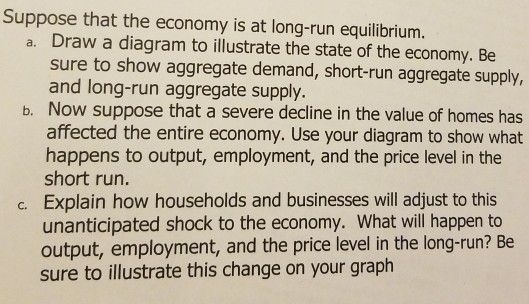Suppose that the economy is at long-run equilibrium. a. Draw a diagram to illustrate the state of the economy. Be sure to show aggregate demand, short-run aggregate supply, and long-run aggregate supply. b. Now suppose that a severe decline in the value of homes has affected the entire economy. Use your diagram to show what happens to output, employment, and the price level in the short run. Explain how households and businesses will adjust to this unanticipated shock to the...

• ### Short Answer 1. Use an aggregate demand / apgregate supply diagram to illustrate at a short...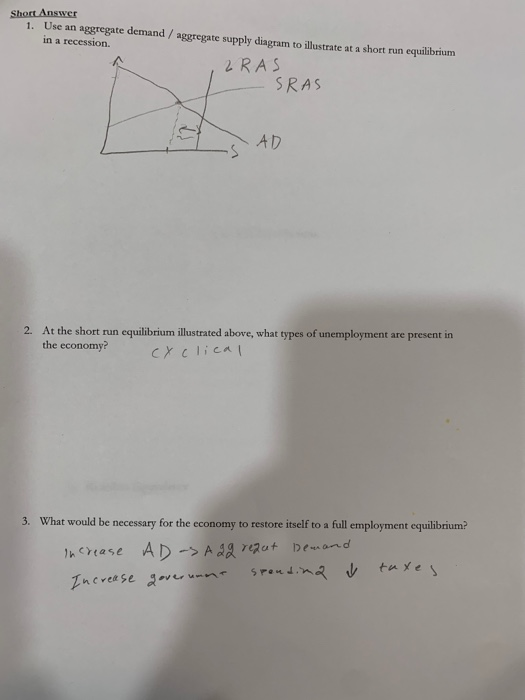Short Answer 1. Use an aggregate demand / apgregate supply diagram to illustrate at a short run equilibrium in a recession 2 RAS - SRAS AD 2. At the short run equilibrium illustrated above, what types of unemployment are present in the economy? CYClien 3. What would be necessary for the economy to restore itself to a full employment equilibrium? increase AD-SA 2g requt Demand Increase government spendina V taxes.

• ### Unit 3: Aggregate Demand, Aggregate Supply, and Fiscal Policy AD, AS, and LRAS Short Run vs....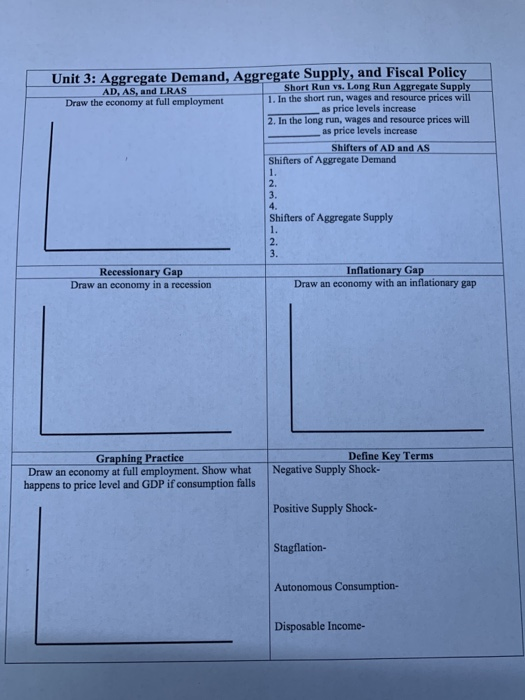Unit 3: Aggregate Demand, Aggregate Supply, and Fiscal Policy AD, AS, and LRAS Short Run vs. Long Run Aggregate Supply Draw the economy at full employment 1. In the short run, wages and resource prices will as price levels increase 2. In the long run, wages and resource prices will as price levels increase Shifters of AD and AS Shifters of Aggregate Demand Shifters of Aggregate Supply imi Recessionary Gap Draw an economy in a recession Inflationary Gap Draw an...

• ### -refully Using aggregate supply and aggregate demand curves, illustrate and summarize what impact each of the...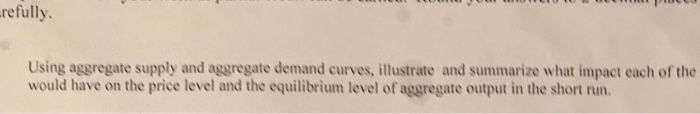-refully Using aggregate supply and aggregate demand curves, illustrate and summarize what impact each of the would have on the price level and the equilibrium level of aggregate output in the short run. During a period of increasing aggregate demand, an economy experiences large gains in productivity.

• ### Draw and carefully describe a graph that utilizes theAggregate Demand/Aggregate Supply model that would illustrate...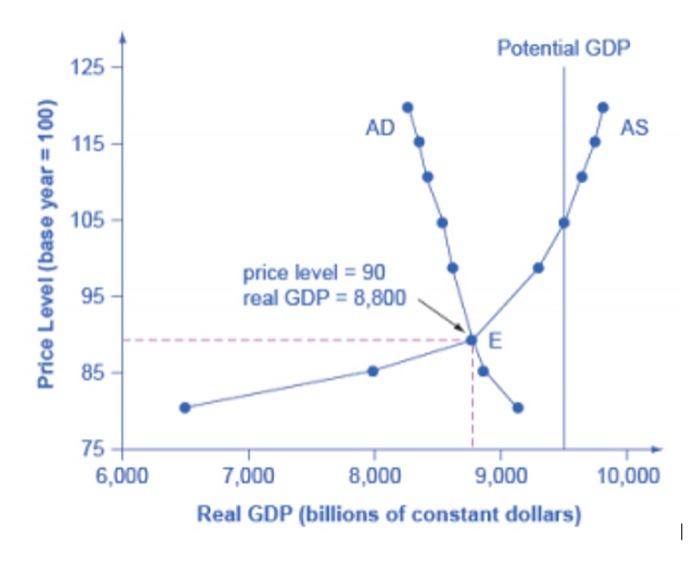Draw and carefully describe a graph that utilizes the Aggregate Demand/Aggregate Supply model that would illustrate the current state of the aggregate economy in the United States. The Aggregate Demand/Aggregate Supply Model is first explained in Chapter 11of your text. Carefully explain your graph.You should draw your own AD/AS graph which you can then scan and paste into your post. Your graph needs to be clearly labeled and explained carefully. Make sure that your graph includes an aggregate demand (AD)...

• ### Using aggregate demand (AD), short-run aggregate supply (SRAS) and long-run aggregate supply (LRAS) curves

Question 1: AD-SRAS-LRAS Model Using aggregate demand (AD), short-run aggregate supply (SRAS) and long-run aggregate supply (LRAS) curves, graphically illustrate the effect of an increase in the money supply on output and prices in the short and long run. Assume that the economy is initially in long run equilibrium at the potential output level and prices are fixed in the short-run. In your graph, label "A" for the initial equilibrium, "B' for the short-run equilibrium, and "C" for the long-run equilibrium.

• ### Long run aggregate supply is the relationship between the quantity of real GDP supplied and the...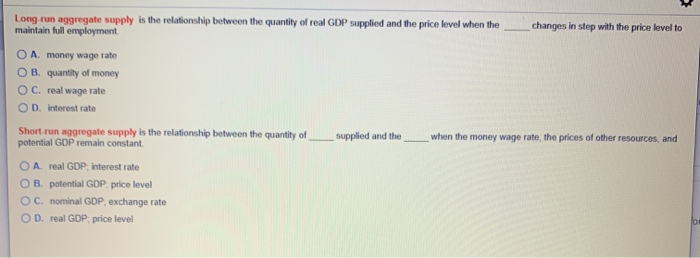Long run aggregate supply is the relationship between the quantity of real GDP supplied and the price level when the maintain full employment changes in step with the price level to O A. money wage rate OB. quantity of money OC. real wage rate OD. interest rate supplied and the when the money wage rate, the prices of other resources and Short run aggregate supply is the relationship between the quantity of potential GDP remain constant O A real GDP...

• ### Draw a basic aggregate demand and aggregate supply graph (with LRAS constant) that shows the economy...

Draw a basic aggregate demand and aggregate supply graph (with LRAS constant) that shows the economy in long-run equilibrium. a. Assume that there is a large increase in demand for U.S. exports. Show the resulting short-run equilibrium on your graph. In this short-run equilibrium, is the unemployment rate likely to be higher or lower than it was before the increase in exports? Briefly explain. Explain how the economy adjusts back to long-run equilibrium. When the economy has adjusted back to...

• ### 165 9. Which of the following should not be considered to be a supply side policy?...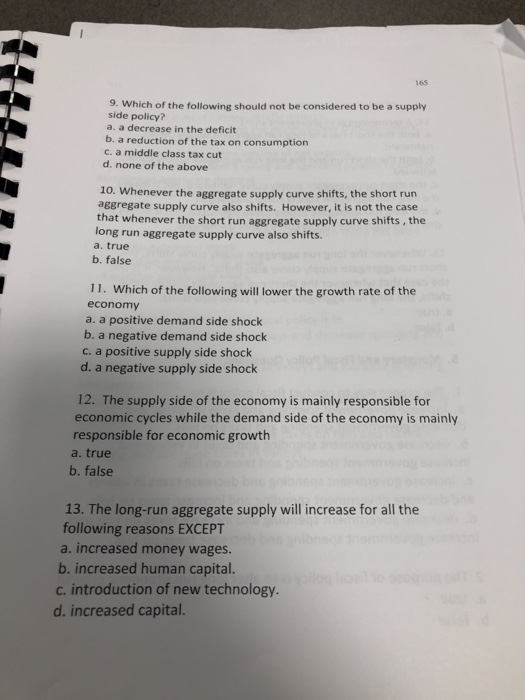165 9. Which of the following should not be considered to be a supply side policy? a. a decrease in the deficit b. a reduction of the tax on consumption c. a middle class tax cut d. none of the above 10. Whenever the aggregate supply curve shifts, the short rur aggregate supply curve also shifts. However, it is not the case that whenever the short run aggregate supply curve shifts, the long run aggregate supply curve also shifts. a....